# Illustrated, 700 pages of machine learning notes are on fire! worth learning

Posted Jun 15, 20204 min read

Recently, I was learning machine learning. I saw this note, which was very detailed and recorded as learning.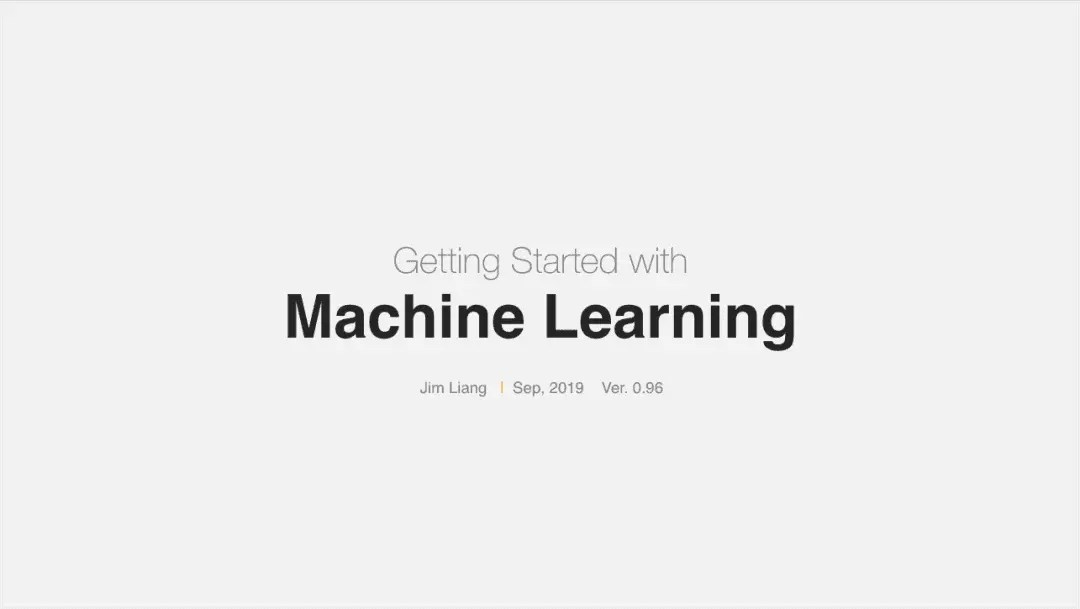# Author

Jim Liang, from SAP(the world's largest business software company).

# Book features

Clearly organized, with graphical representations that are easier to understand, with detailed notes on formulas, etc.

# abstract

Mainly divided into basic concepts, commonly used algorithms and other three parts.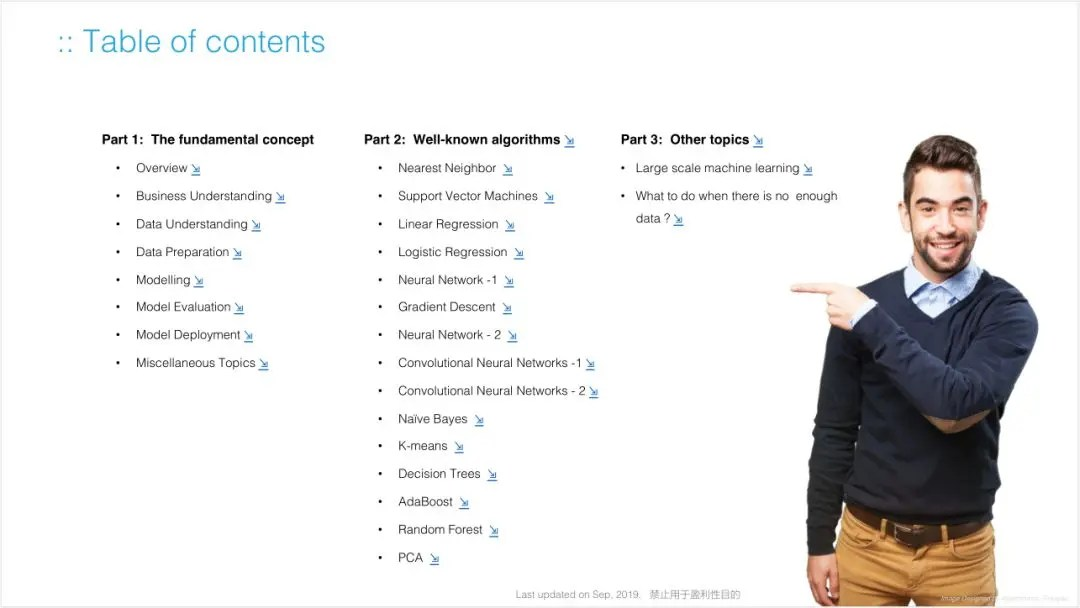Why is this happening?

• The first one is mathematics, which involves statistics, calculus, probability, linear algebra, etc. Although everyone has studied advanced mathematics, if you still remember the details inside, it is your cattle. It is more likely that most people have forgotten about advanced mathematics and are disgusted or even afraid of the large number of formulas in various algorithms.
• Secondly, because machine learning itself is a comprehensive subject and a fast-developing subject, knowledge points are scattered and lack of systematization.
• Machine learning/deep learning books, articles, and tutorials on the market are blossoming everywhere, but there are not many tutorials that can be expressed in a clear way and explained step by step. In fact, there are not many tutorials. A large number of tutorials do not consider the foundation of the learner, making beginners feel Frustrated and confused.
• It is the experience of the pain in the process of machine learning. The author Jim Liang hopes to do a tutorial to explain it in a simple and understandable way to lower the learning threshold for everyone. It took me several months to do this, often late at night, and organized my study notes into this tutorial.

# Part 1 introduces basic concepts, including:

• Machine learning process
• data processing
• Modeling
• Evaluation index(such as MSE, ROC curve)
• Model deployment
• Overfitting
• Regularization, etc.

In the first part, the author first introduces the machine learning that is commonly used today:from autonomous driving, voice assistants to robots. Some of these ideas are also understood by many readers, for example:why machine learning is hot at this time(big data, computing power, better algorithms); the relationship between machine learning, artificial intelligence, and deep learning.

In addition to these basic concepts, this tutorial also graphically displays the development process of the machine learning model(as shown below). Even readers who do not understand this can learn through this process.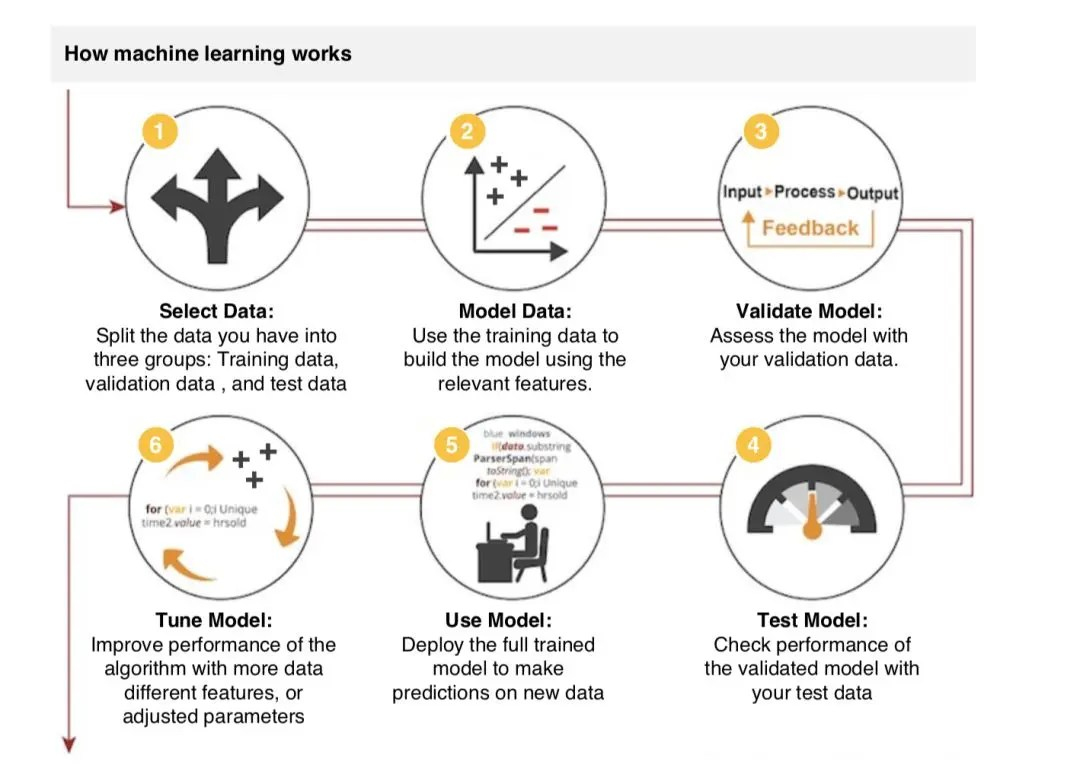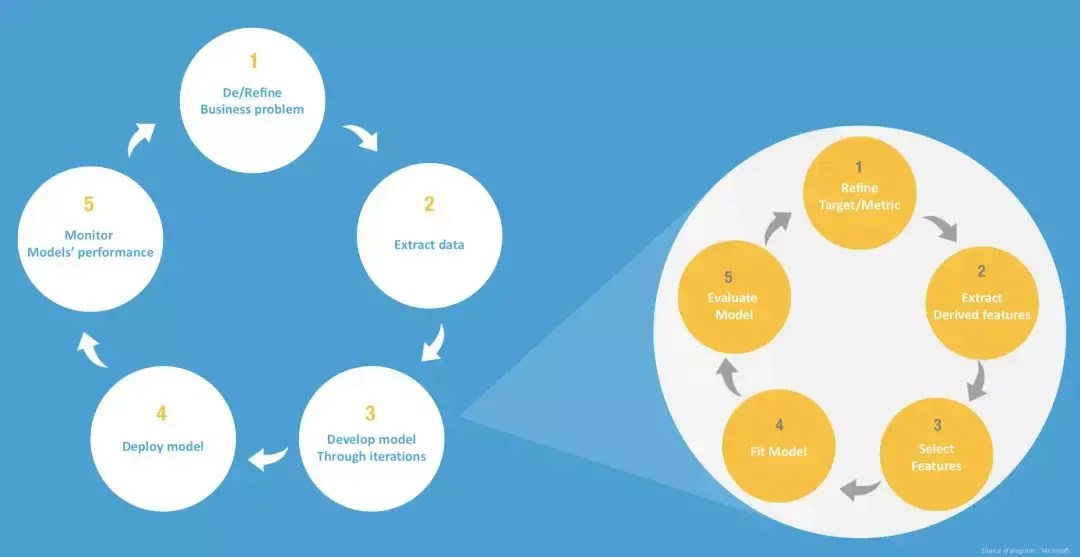# Electronic version of 700 pages of machine learning notes:

Public number [Computer Vision Alliance]Backstage reply:9001, you can get the electronic version

# In Part2, the author introduced commonly used algorithms, including:

• Linear regression
• Logistic regression
• Neural Networks
• SVM
• Knn
• K-Means
• Decision tree
• Random forest
• Naive Bayes
• Principal component analysis

This part contains a lot of mathematical formulas, but the author tries his best to annotate each of them, so as to fully and clearly express many mathematical concepts.

For example, in the "Neural Network" section, the author organized 59 pages of notes(from pages 311 to 369). The author starts with the neuron architecture in the human brain and introduces the working principles of artificial neural networks(ANN) and artificial neurons. This note is very focused on the conceptual explanation of the image, and it is very intuitive to understand.

For example, the conceptual explanation in the figure below vividly shows the similarity in the way biological neurons and artificial neurons work.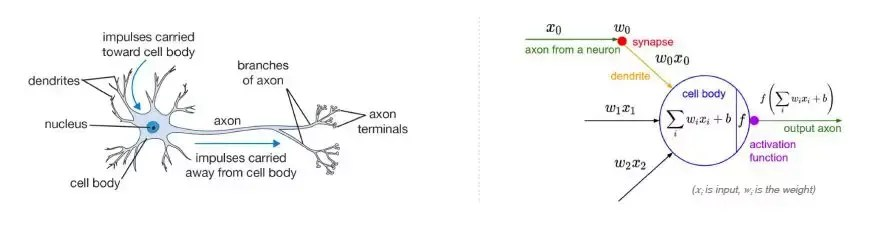Comparison of the dendrite input-axon output mode of biological neurons and the input and output mode of artificial neurons.

When it comes to mathematical formulas, the author will have detailed notes next to it, as shown in the following figure:For the parallel options(such as activation function, common neural network architecture, etc.), there will also be a comprehensive list:

For more complex concepts in neural networks(such as derivation, back propagation), a few pictures can explain clearly:In order to facilitate everyone's learning, we have prepared a full version of the machine learning notes PDF. Interested students can easily obtain them by following the steps below:

# Electronic version of 700 pages of machine learning notes:

Public number [Computer Vision Alliance]Backstage reply:9001, you can get the electronic version